# Combo Calculator 1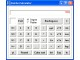Combo Calculator is a full featured free calculator providing all the essential features of a calculator.

 Developer: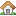1888softwaredownloads      software by 1888softwaredownloads → Price: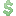0.00 License: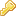Freeware File size:0K Language: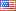OS: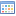Windows Vista (?) Rating: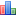0 /5 (0 votes)

Combo Calculator is a full featured free calculator providing all the essential features of a calculator.

The software handles all basic and advanced features required by most users and includes several advanced features within a scientific calculator.

Combo Calculator evaluates all major mathematical expressions and supports various trigonometric functions, exponential function, logarithms, and factorials. Round and cube root are the special features of this calculator.

tags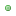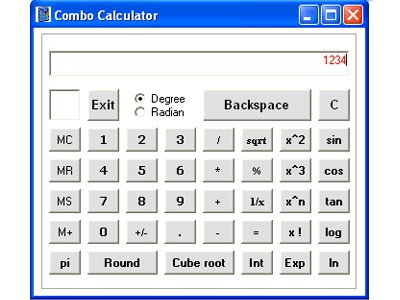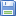Download Combo Calculator 1

###### Similar softwareCombo Calculator 1

Combo Calculator is a full featured free calculator providing all the essential features of a calculator.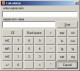Free Calculator 1.0

Free Calculator is a useful calculator that can perform any of the standard operations for which you would normally use a handheld calculator.RJ Calculator 2.71
rjsoftware

RJ Calculator is a scientific calculator with most basic functions like trigonometric functions, logarithms and base conversion.Aepryus Calculator 1.2
Aepryus Software

Aepryus Calculator is a scientific RPN calculator.DeadLine Calculator 1.0
Ionut Alex. Chitu

Designed especially for students and engineers, DeadLine Calculator is an advanced command-line calculator useful if you want to evaluate complicated expressions in a simple way.Calculator Pro 2.0.042
Armin Richter

Calculator Pro is a calculator tool for Windows similar to common pocket calculator.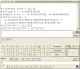FAS Calculator 2.0.0.1
FreeART Software

FAS Calculator is an expression calculator which allows you to directly enter an expression to be evaluated.Calculator (Basic)
Vedran

Calculator is a free and useful utility which is used for advanced calculations.PG Calculator 2.2
Piotr Gridniew

PG Calculator is a scientific multifunction skinable calculator that operates in three working modes: standard, algebraic and RPN.Console Calculator 2.2.0
Scott Cogan

Console Calculator quickly evaluates simple and advanced mathematical expressions, remembers recent entries, allows creation of user defined variables and custom functions.